# Topological space

Jump to: navigation, search
This article is about a basic definition in topology.
VIEW: Definitions built on this | Facts about this | Survey articles about this
View a complete list of basic definitions in topology

## Definition

A topological space is a set endowed with a topology. A topology on a set$S$ is a collection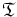$\mathfrak{T}$ of subsets of the set, called open subsets, satisfying the following:

• The empty set and the whole space are in$\mathfrak{T}$
• An arbitrary union of members of$\mathfrak{T}$ is in$\mathfrak{T}$
• A finite intersection of members of$\mathfrak{T}$ is in$\mathfrak{T}$

When we want to emphasize both the set and its topology, we typically write them as an ordered pair. So, a set$S$ with a topology$\mathfrak{T}$ is denoted$(S,\mathfrak{T})$. Usually, when the topology is understood or pre-specified, we simply denote the topological space by its underlying set,$S$.

## Notation and terminology

### Elements of the topological space

The elements of the underlying set of a topological space are termed points, and are typically denoted by lower case letters. Typical letters used for points include$p,q,x,y$, though other letters are also often used.

### Subsets of the topological space

Subsets of a topological space are typically denoted by capital letters. Open subsets are usually denoted by letters like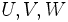$U, V, W$, while closed subsets are typically denoted by letters like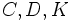$C,D,K$ (the letters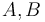$A,B$ are used both for open and for closed subsets).

A subset of a topological space can naturally be given a topology: the so-called subspace topology, and the same letter is used for it, both as a subset and as a subspace with the subspace topology.

### Indexing sets

It is frequent in topology to consider collections of subsets, and the typical notation used is that of an indexing set. Indexing sets are typically denoted by letters like$I$ and$J$. Thus, a collection of open subsets of a topological space$S$ might be denoted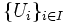$\{ U_i \}_{i \in I}$ where$I$ is an indexing set. When the indexing set is finite, we can choose it to be$\{ 1,2, \dots, n \}$, and the collection becomes$\{ U_1, U_2, \dots, U_n \}$.

## Examples

### Extreme examples

The two extreme examples of a topological space are:

1. The discrete space: This is a topological space where every subset is open. A topology on a set where every subset is open is termed the discrete topology.
2. The trivial topology is the topology where the only open subsets are the empty subset and the whole space

### Occurrence of topological spaces

Topological spaces occur in many situations, typically arising from other kinds of sets with additional structure. Here are some examples:

• Euclidean space, i.e., the space$\R^n$, comes with a natural topology, where a subset$U$ of$\R^n$ is termed open if, for every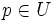$p \in U$, there exists a ball centered at$p$, that lies completely inside$U$
• sub-Euclidean space: Any subset of Euclidean space can be given a topology in a similar fashion. If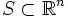$S \subset \R^n$, then a subset$U$ of$S$ is termed open in$S$ if there exists a ball centered at$p$ whose intersection with$S$ lies completely inside$U$
• Any metric space gets a natural topology, with the notion of ball being replaced by a metric ball
• Any simplicial complex gives rise to a topological space, the so-called geometric realization.

## Constructions

### Open and closed subsets

Further information: Open subset,closed subset The definition of a topological space includes a specification of what subsets of the topological space are open. A closed subset of a topological space is defined as a subset whose set-theoretic complement in the space is open. In other words, if$X$ is a topological space and$U$ is an open subset of$X$, then$X \setminus U$ is termed a closed subset.

### Basis of a topological space

Further information: Basis of a topological space

Given a topological space$X$, a basis for$X$ is a collection of open subsets of$X$ with the property that every open subset of$X$ can be expressed as a union of some members of the collection. The typical example of a basis is the open balls in$\R^n$: every open subset of$\R^n$ is a union of open balls.

Given a set without a topology, we can take a collection of subsets and ask whether that collection of subsets can occur as the basis of some topology. For this, the collection of subsets must satisfy a condition. Modulo that condition, the basis completely determines the topology.

### Subbasis of a topological space

Further information: Subbasis of a topological space

Given a topological space$X$, a subbasis for$X$ is a collection of open subsets such that, starting from that collection, and doing operations of finite intersections and arbitrary unions, we can get every open subset of$X$.

Again, we can specify a topology on a set using a subbasis for it. Further, unlike a basis, a subbasis need not satisfy any condition: we can start with any collection of subsets of a topological space, and we get a topology with that collection of subsets as a subbasis.

### Continuous map of topological spaces

Further information: Continuous map of topological spaces